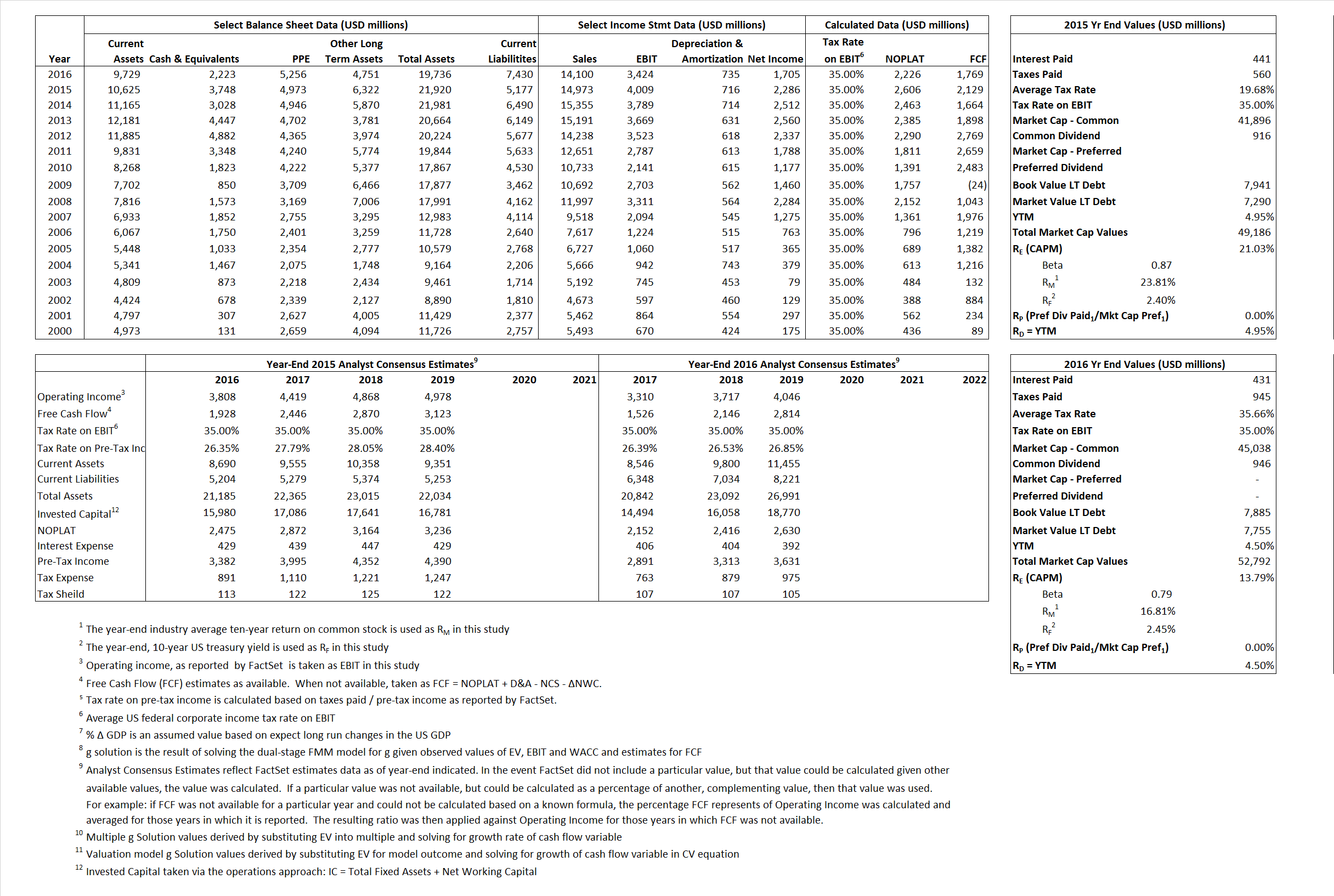# Monsanto

## Analyst Listing

The following analysts provide coverage for the subject firm as of May 2016:

 Broker Analyst Analyst Email Piper Jaffray Brett Wong brett.ws.wong@pjc.com Monness Crespi Hardt Chris L. Shaw cshaw@mchny.com BB&T Capital Markets Christopher J. Kapsch ckapsch@bbandtcm.com Credit Suisse Christopher S. Parkinson christopher.parkinson@credit-suisse.com Atlantic Equities Colin Isaac c.isaac@atlantic-equities.com Deutsche Bank Research David Begleiter david.begleiter@db.com Susquehanna Financial Group Don Carson don.carson@sig.com Wells Fargo Securities Frank J. Mitsch frank.mitsch@wellsfargo.com BMO Capital Markets Joel Jackson joel.jackson@bmo.com Bernstein Research Jonas Oxgaard jonas.oxgaard@bernstein.com Jefferies Laurence Alexander lalexander@jefferies.com Stifel Nicolaus Paul Massoud pamassoud@stifel.com

## Primary Input Data## Derived Input Data

### Equational Form

Net Operating Profit Less Adjusted Taxes NOPLAT 2,606 2,226$NOPLAT\, =\, EBIT\, x\, (1 \,-\, Avg \,\,Tax\,\, Rate\,\, on\,\, EBIT)$
Free Cash Flow FCF 2,129 1,769$FCF\,=NOPLAT\,+\,Non-Cash\,Expenses-\Delta NWC\,-\,NCS$
Tax Shield TS 87  154$TS\,=\,Interest\,\,Paid\,\,x\,\, Avg \,\,Tax\,\,Rate\,\, on\,\, Pre-Tax\,\, Income$
Invested Capital IC 16,743 12,306$IC\,=\,Fixed\,\,Operating\,\,Assets\,\,+\,\,Net\,\, Working\,\, Capital$
Return on Invested Capital ROIC 15.56%  18.09%$ROIC\,=\,\frac { NOPLAT }{ IC }$
Net Investment NetInv  1,968 (3,702)$NetInv\,=\,{ {IC}_{1}}-{{IC}_{0}}+Depreciation$
Investment Rate IR 75.52% -166.34%$IR\,=\,\frac {NetInv}{NOPLAT}$
Weighted Average Cost of Capital
WACCMarket  18.50%  12.19%$WACC\,=\,\frac { E }{ V } { R }_{ E }\,+\,\frac { P }{ V } { R }_{ P }\,+\,\frac { D }{ V } { R }_{ D }\left( 1- Avg\,\, Tax\,\,Rate\,\,on\,\,Pre-Tax\,\,Income \right)$
WACCBook  6.97%    6.71%
Enterprise value
EVMarket 45,438  50,569$EV\,=\,Market\,\,Cap\,\,Equity\,+\,\,Long\,\,Term\,\,Debt\,-\,Cash$
EVBook  47,614    50,700
Long-Run Growth
g = IR x ROIC
11.75%  -30.08% Long-run growth rates of the income variable are used in the Continuing Value portion of the valuation models.
g = %$\Delta$ GDP  2.50%    2.50%
Margin from Operations M  26.77%  24.28%$M\,\,=\,\,\frac{EBIT}{SALES}$
Depreciation/Amortization Rate D  15.15%  17.67%$D\,\,=\,\,\frac{D+A}{EBITDA}$

## Valuation Multiple Outcomes

The outcomes presented in this study are the result of original input data, derived data, and synthesized inputs.

### model g solution

12/31/2015 12/31/2016 12/31/2015 12/31/2016 12/31/2015 12/31/2016

EV/SALES$\frac {EV}{Sales} \,= \,\frac{ROIC\, -\, g}{ROIC\,(WACC\,-\,g)}\,(1\,-\,T)\,(M)$

3.03  3.59  20.21%  10.30%  19.32%  10.97%

EV/EBITDA$\frac {EV}{EBITDA} \,= \,\frac{ROIC\, -\, g}{ROIC\,(WACC\,-\,g)}\,(1\,-\,T)\,(1\,-\,D)$

9.62 12.16 20.21% 10.30% 19.32% 10.97%

EV/NOPLAT$\frac {EV}{NOPLAT} \,= \,\frac{ROIC\, -\, g}{ROIC\,(WACC\,-\,g)}$

17.44 22.72 20.21% 10.30% 19.32% 10.97%

EV/FCFOPS$\frac {EV}{FCF_{OPS}} \,= \,\frac{ROIC\, -\, g}{ROIC\,(WACC\,-\,g)}\,(1\,-\,T)$

21.35 28.59 20.21% 10.30% 19.32% 10.97%

EV/EBIT$\frac {EV}{EBIT} \,= \,\frac{ROIC\, -\, g}{ROIC\,(WACC\,-\,g)}\,(1\,-\,T)$

11.33  14.77 20.21% 10.30% 19.32% 10.97%

EV/IC$\frac {EV}{IC} \,= \,\frac{ROIC\, -\, g}{WACC\,-\,g}$

2.71 4.11 20.21% 10.30% 19.32% 10.97%